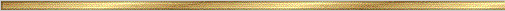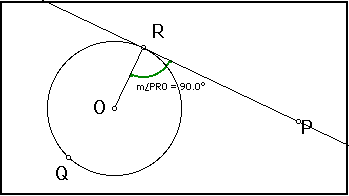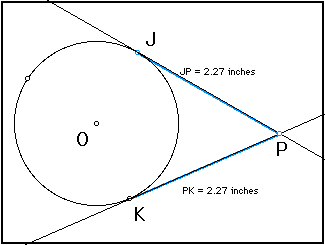# Tangent Conjectures### Explanation:

A tangent line to a circle is any line which intersects the circle in exactly one point. You can think of a tangent line as "just touching" the circle, without ever traveling "inside". A line which intersects a circle in two points is called a secant line. Chords of a circle will lie on secant lines.

### The precise statement of the conjecture is:

Conjecture (Tangent Conjecture I ): Any tangent line to a circle is perpendicular to the radius drawn to the point of tangency.Conjecture (Tangent Conjecture II ): Tangent segments to a circle from a point outside the circle are equal in length.• Key Curriculum Press can provide demo versions of Geometer's Sketch Pad